﻿ 考虑共同关注区域静态交谈群组检测的机器人导航及行为评价
 机器人2022, Vol. 44Issue (4): 494-5030

ZHOU Lei, ZHAO Kunxu, SONG Yinuo, LIU Jingtai. Detecting Static Conversation Group with Common Concern Area for Robot Navigation and Behavior Evaluation[J]. ROBOT, 2022, 44(4): 494-503.1. 南开大学人工智能学院机器人与信息自动化研究所, 天津 300350;
2. 天津市智能机器人技术重点实验室, 天津 300350

Detecting Static Conversation Group with Common Concern Area for Robot Navigation and Behavior Evaluation
ZHOU Lei1,2 , ZHAO Kunxu1,2 , SONG Yinuo1,2 , LIU Jingtai1,21. Institute of Robotics and Automatic Information System, College of Artificial Intelligence, Nankai University, Tianjin 300350, China;
2. Tianjin Key Laboratory of Intelligent Robotics, Tianjin 300350, China
Abstract: An F-formation detection algorithm based on common concern areas is proposed for static conversation group. It takes the position and direction of pedestrians as input to construct the common concern area of groups. Then a sliding window-based maximum filter is used to detect the group center for clustering. After detecting the static conversation group, a group comfort space is constructed for a time-dependent A* path planning algorithm based on multi-layer costmap mechanism. Thus, mobile robot navigation considering group comfort is realized. In addition, it is challenging to quantitatively evaluate whether a robot's navigation behavior is socially acceptable. A graph convolutional network-based model for evaluating robot behavior is constructed. Experimental results show that the evaluation network has a similar capacity to humans in evaluating the robot behavior. Visualization results show the rationality of the evaluation network's results. According to the evaluation network, robots considering static conversation group can produce more comfortable trajectories.
Keywords: static conversational group detection    F-formation    common concern area    group comfort space    social aware robot navigation    graph convolutional network

1 引言（Introduction）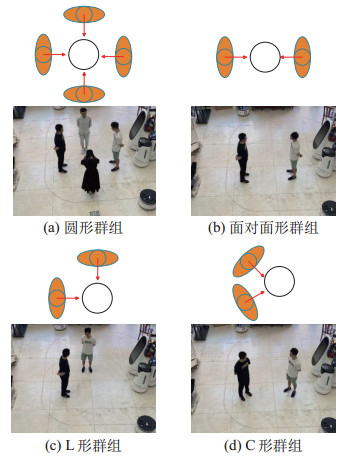图 1 典型的F型社交关系构形 Fig.1 Typical F-formation configurations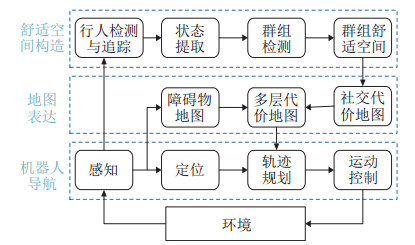图 2 考虑F型社交关系的机器人导航框架 Fig.2 F-formation aware robot navigation framework
2.1 状态表示

 \begin{align} h_{i} =\{{x_{i}, y_{i}, \theta_{i}}\} \end{align} (1)

 \begin{align} f_{i} (x, y)=\;& \exp \{ -[a({x-\tilde{x}_{i}})^{2}-2b({x-\tilde{x}_{i}})({y-\tilde{y}_{i}}) + \\ & c({y-\tilde{y}_{i}})^{2}]\} \end{align} (2)

 \begin{align} \begin{aligned} a & =\frac{\cos^{2}\theta_{i}} {2\sigma_{x}^{2}} +\frac{\sin^{2}\theta_{i}} {2\sigma_{y}^{2}} \\ b & =\frac{\sin^{2}2\theta_{i}} {4\sigma_{x}^{2}} +\frac{\sin^{2}2\theta_{i}} {4\sigma_{y}^{2}} \\ c & =\frac{\sin^{2}\theta_{i}} {2\sigma_{x}^{2}} +\frac{\cos^{2}\theta_{i}} {2\sigma_{y}^{2}} \end{aligned} \end{align} (3)

$(\tilde{x}_{i}, \tilde{y}_{i})=(x_{i}, y_{i})$表示人的舒适空间中心点；$(\tilde{x}_{i}, \tilde{y}_{i})=({x_{i} +r\cos \theta_{i}, y_{i} +r\sin \theta_{i}})$表示人的关注区域中心点。参数$r $$\sigma_{x}$$ \sigma_{y}$控制着对应区域的大小，将其都设置为个人舒适空间大小的一半（0.6 m），其中\sigma_{x} \sigma_{y} 可以通过个人相关的特质进行调整，这部分研究内容在之前已经进行了研究，这里不再赘述。 2.2 静态交谈群组检测 关于静态群组的检测，在机器人领域与计算机视觉领域都进行了大量的研究。但是先前的工作[15-18]大多基于数据驱动，采用机器学习或者深度学习的方式通过大量的数据训练得到。与此不同的是，本文利用之前所提的共同关注区域的概念，通过共同关注区域提取群组中心点进行群组检测。该方法无需数据训练，仅需手动调节少量参数即可完成静态交谈群组的检测。 在获得个人关注区域的表示之后，通过将所有人的关注区域进行叠加可以考虑环境中所有人：  \begin{align} f(x, y)=\sum _i f_{i} (x, y) \end{align} (4) f(x, y) 表示环境整体的共同关注区域的代价累加值，对应的等高线图如图 3所示。图 3 共同关注区域等高线图 Fig.3 The contours of common concern area 图 3为典型F型社交关系的静态交谈群组共同关注区域的等高线图，其中等高线中心蓝色的点表示群组的中心。在图 3所示的关注区域图上采用左上角的滑动窗口对关注区域图进行遍历，在遍历过程中通过最大值滤波法提取局部最大值，然后将滑动窗口的中心值与滑动窗口范围内的其他区域进行比较，当滑动窗口的中心值比窗口区域内的其他值大时，滑动窗口中心即为群组中心 ({x_{{\rm g}_{n}}, y_{{\rm g}_{n}}}) 。群组中心被存入一个数组 C_ G =\{(x_{{\rm g}_1}, y_{{\rm g}_1}), ({x_{{\rm g}_{2}}, y_{{\rm g}_{2}}}), \cdots, ({x_{{\rm g}_{n}}, y_{{\rm g}_{n}}})\} 中。在得到群组中心之后，将式(2) 修改为 (\tilde{x}_{i}, \tilde{y}_{i})=(x_{{\rm g}_{n}}, y_{{\rm g}_{n}}) ，然后采用算法1对属于同一群组的人进行聚类，其中 f_{\rm{th}} 为超参数，需要根据实验场景进行调节。  算法2 静态交谈群组检测算法 输入: 环境中所有人的位置 { P} ，超参数 f_{\rm th} ，群组中心 { C_{G}} 输出: 群组集合 { G} 1: for { c_g} \in { C_{G}} do 2: 初始化一个空群组 { g} 3: for { h_i} \in { H} do 4: 利用式(2) 计算 f_i({ c_{g_x}}, { c_{g_y}}) 5: if f_i({ c_{g_x}}, { c_{g_y}}) > f_{\rm th} then 6: 将 { h_i} 加入群组 { g} 7: end if 8: end for 9: 将群组 { g} 加入群组集合 { G} 10: end for 11: return { G} 2.3 群组舒适空间 在完成静态交谈群组检测之后，根据文[11-12]构造群组舒适空间。文[11-12]对群组舒适空间进行了描述，群组的舒适空间并不是群组个体舒适空间简单的叠加，群组的成员在维护自身舒适空间的同时也维护着共同关注区域的舒适度，其他人闯入共同关注区域也会引起群组成员的不适。将群组中心视为共同关注区域的中心，并且使用扩展混合高斯模型构造群组舒适空间 F_{{\rm g}_{n}}  \begin{align} F_{{\rm g}_{n}} =\sum _m {w_{m}} f_{{\rm h}_{i}} (x, y)+w_{\rm c} f_{\rm c} (x_{{\rm g}_{n}}, y_{{\rm g}_{n}}) \end{align} (5) 其中 f_{{\rm h}_{i}} (x, y) 表示个人的舒适空间， f_{\rm c} (x_{{\rm g}_{n}}, y_{{\rm g}_{n}}) 表示群组的共同关注区域， w_{m} w_{\rm c}分别表示个人舒适空间与群组舒适空间所占的权重，本文都取1。

2.4 地图表达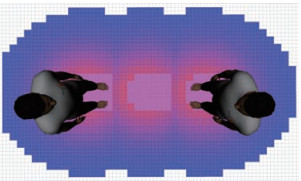图 4 社交代价地图 Fig.4 Social costmap
2.5 机器人导航

 \begin{align} U=\{{({e_{x} a_{x}, e_{\phi} a_{\phi}})\mid e_{x}, e_{\phi} \in \{-1, 0, 1\}}\} \end{align} (6)

 \begin{align} \mathcal{C}=\sum _i {w_{i}} \cdot c_{i} \end{align} (7)

3 机器人行为评价网络（Robot behavior evaluation network）

3.1 基于图的状态表示

3.2 关系推理

 $\begin{equation} \mathit{\boldsymbol{r}} =[0, 0], \quad \mathit{\boldsymbol{h}} =[x, y, \cos \theta, \sin \theta] \end{equation}$ (8)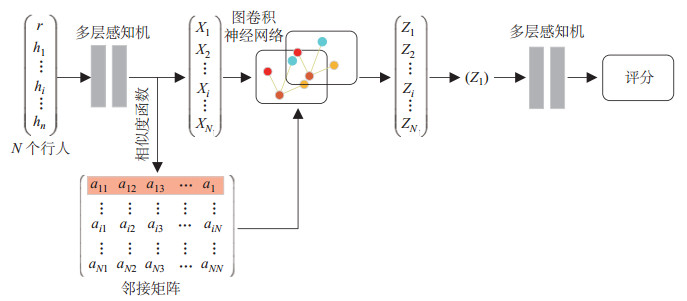图 5 基于图卷积网络的机器人行为评估网络 Fig.5 GCN-based robot behavior evaluation network

1) 高斯函数：

 \begin{align} f({\mathit{\boldsymbol{x}}_{i}, \mathit{\boldsymbol{x}}_{j}})={\rm e}^{ {\mathit{\boldsymbol{x}}_{i}^{\rm T}}{\mathit{\boldsymbol{x}}_{j}}} \end{align} (9)

2) 嵌入式高斯函数。高斯函数的一个扩展，可用于计算嵌入空间的相似度：

 \begin{align} f({\mathit{\boldsymbol{x}}_{i}, \mathit{\boldsymbol{x}}_{j}})={\rm e}^{\theta ({\mathit{\boldsymbol{x}}_{i}^{\rm T}})\phi ({\mathit{\boldsymbol{x}}_{j}})} \end{align} (10)

3) 余弦函数。余弦相似度为

 \begin{align} f({\mathit{\boldsymbol{x}}_{i}, \mathit{\boldsymbol{x}}_{j}})=\frac{{\mathit{\boldsymbol{x}}_{i}^{\rm T}}{\mathit{\boldsymbol{x}}_{j}}}{|\mathit{\boldsymbol{x}}_{i} ||\mathit{\boldsymbol{x}}_{j} |} \end{align} (11)

4) 余弦函数+归一化指数函数。在余弦相似度的结果上按行通过softmax函数归一化。

5) 均等注意力函数。所有智能体之间的重要程度相等，即：

 \begin{align} f({\mathit{\boldsymbol{x}}_{i}, \mathit{\boldsymbol{x}}_{j}})=\frac{1}{{ {N}}} \end{align} (12)

 \begin{align} {\mathit{\boldsymbol{A}}}={\rm softmax} ({{\mathit{\boldsymbol{XW}}}_{a} {\mathit{\boldsymbol{X}}}^{\rm T}}) \end{align} (13)

{\mathit{\boldsymbol{W}}}_{a} ={\mathit{\boldsymbol{W}}}_{\theta} {\mathit{\boldsymbol{W}}}_{\phi}^{\rm T} {\mathit{\boldsymbol{W}}}_{a} 表示需要学习的参数矩阵， {\mathit{\boldsymbol{A}}} 表示学习得到的邻接矩阵，对其进行了按行归一化处理。 3.3 节点消息传递 给定特征矩阵 {\mathit{\boldsymbol{X}}} 与邻接矩阵 {\mathit{\boldsymbol{A}}} 之后，使用多层图卷积网络将人的状态信息在图结构上进行信息传递和向量融合，节点之间的消息传递规则表示为  \begin{align} {\mathit{\boldsymbol{H}}}^{(l+1)}=\sigma ({{\mathit{\boldsymbol{AH}}}^{(l)}{\mathit{\boldsymbol{W}}}^{(l)}})+{\mathit{\boldsymbol{H}}}^{(l)} \end{align} (14) 节点 i 通过存储在邻接矩阵 {\mathit{\boldsymbol{A}}} 中的关系权重来聚合第 l 层的节点特征，从而获得自身在第 l+1 层的表示。 {\mathit{\boldsymbol{W}}}^{(l)} 是第 l 层的网络权重，每层图卷积网络后面都增加了一个ReLU（线性整流）的激活函数 \sigma {\mathit{\boldsymbol{H}}}^{(l)} 是第 l 层图卷积网络的输出特征，其中初始输入 {\mathit{\boldsymbol{H}}}^{(0)} 被设置为特征矩阵 {\mathit{\boldsymbol{X}}} {\mathit{\boldsymbol{Z}}}表示最后一层图卷积网络的输出，${\mathit{\boldsymbol{Z}}}[i, :]$表示聚集了周围节点信息的节点$i$的特征。

3.4 基于节点特征的评分网络

 \begin{align} S=\alpha ({\mathit{\boldsymbol{Z}}}_{1}, {\mathit{\boldsymbol{W}}}_{\alpha}) \end{align} (15)

4 实验与分析（Experiments and analysis）

4.1 评分网络实验 4.1.1 模型设置

4.1.2 实验数据与环境

1) 人：包括行人编号、位置、朝向角。

2) 机器人：由于场景是以机器人为参考坐标系构建的，所有机器人在数据集当中仅表示该场景存在机器人。

3) 房间：一系列顶点，表示房间各个墙壁的起点与终点。

4) 物体：一系列位置方向数据，表示边长为40 cm的家具的位姿。

5) 连接关系：一系列人—物的编号，表示人—人、人—物之间是否存在交互。

6) 分数：当前场景的分数在0~100之间。分数越低表示机器人的行为越使人不适。

4.1.3 实验结果与分析表 1 不同相似度函数的训练结果 Tab. 1 The training results based on different similarity functions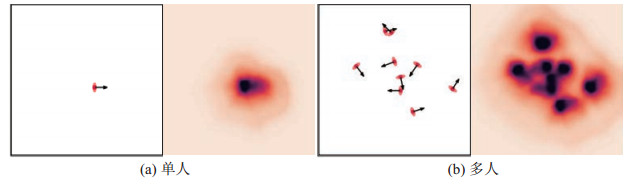图 6 机器人在给定场景下的不适感评分热图 Fig.6 Heatmap of discomfort score for robot in the environment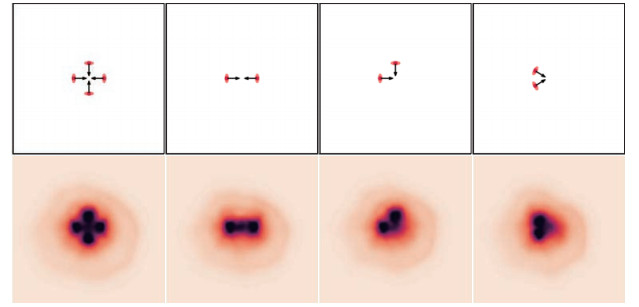图 7 具有F型社交关系构形的群组的不适感评分热图 Fig.7 Heatmap of discomfort score for group with F-formation configuration
4.2 导航实验

4.2.1 仿真环境表 2 代价函数权重 Tab. 2 The weights of cost function
4.2.2 实验结果与分析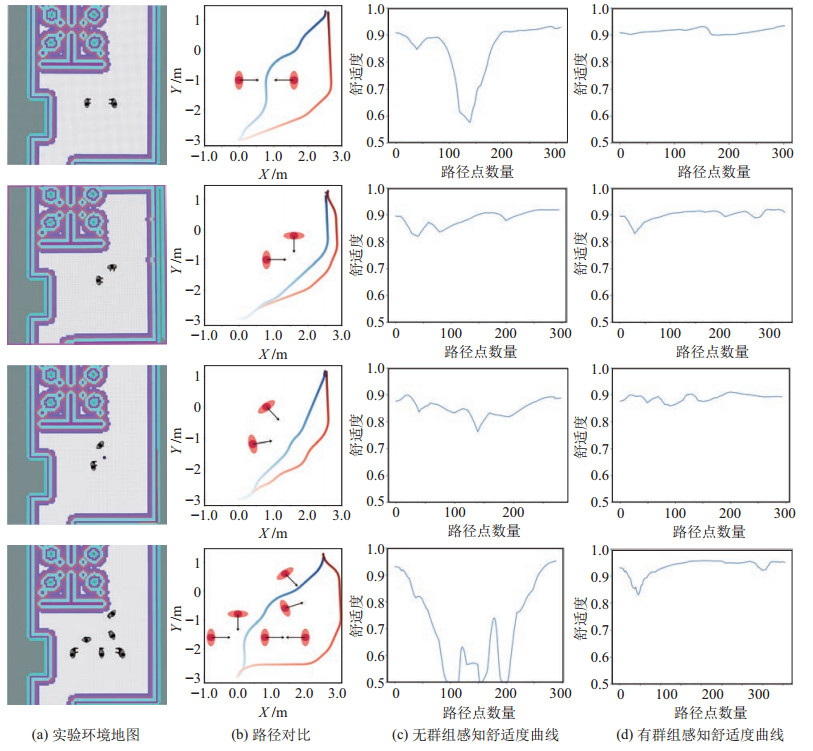图 8 导航实验结果 Fig.8 Results of navigation experiments表 3 舒适度指标对比结果 Tab. 3 Comparison results of comfort index
5 结论（Conclusion）